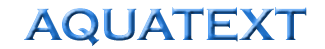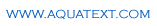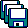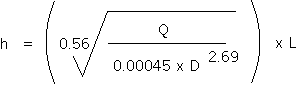The Free On-line Aquaculture DictionaryFriction Loss - Water Pipes Channels

1. Pipes carrying water (smooth bore pipes)A spreadsheet is available for download here which will perform the friction loss calculation for you.

To calculate the flow down a pipe, knowing the headloss : Q = 0.00045 x D2.69 x H0.56

Where Q = flow in litres per second

D - Internal diameter of pipe in millimetres

H = Hydraulic gradient = Metres headloss of pipe (h)

Metres of pipelength (L)

Changing this equation round, the headloss can be calculated if the flow required is known;This is fine for individual lengths of pipe with no fittings, but pipe system are rarely like this, the table below gives an equivalent pipe length to each fitting, and by adding the sum of all the “equivalent lengths” form the fittings and the total pipe length, the above equation can be used to calculate the friction loss (or headloss):

 Fitting Equivalent Pipe Length e.g. 30D = 30 x diameter of pipe 90 degree elbow 30D 45 degree elbow 20D T straight through 16D T through side 60D Swept 90 bend 4-8D Open gate valve 9D Open globe valve 275D Full bore non return valve 6D Butterfly valve 20D
 There are also other methods for calculating friction losses in pipes but the above is the simplest. The headloss figure that is gained form the above calculation can then be used to specify pumps, or the height of the header tank required.
 2. Channels The amount of water which flows down a channel is a function of the cross sectional area, the wetted perimeter, the water velocity, the gradient of the channel and the coefficient of roughness of the channel. Coefficient of roughness of common materials Glazed and very smooth surfaces 0.010 Cement plaster, iron and other smooth pipes in good condition 0.011 Concrete sewers >1500mm diameter 0.012 Concrete sewers 600 - 1500mm diameter 0.013 Brick sided, concrete based channels 0.015 Coarse brick, earth in good order channels 0.020 Earth canal in reasonably good condition, free of stones and weed 0.025 Earth canal in poor condition, with some stones and weed 0.030 Calculation  Q = (1/n x ((A x WP)2/3) x S1/2) x A Where: Q = Flow rate m3/sec n = Coefficient of roughness  (see above) A = Cross sectional area of channel (m2) WP = Wetted perimeter of channel (m) S = Gradient of channel (m). Metres per 100m. e.g. if the channel falls        0.5m every 100m, S=0.5   See also Channel, Limiting velocity and Scouring velocity for other design criteria which must be considered.